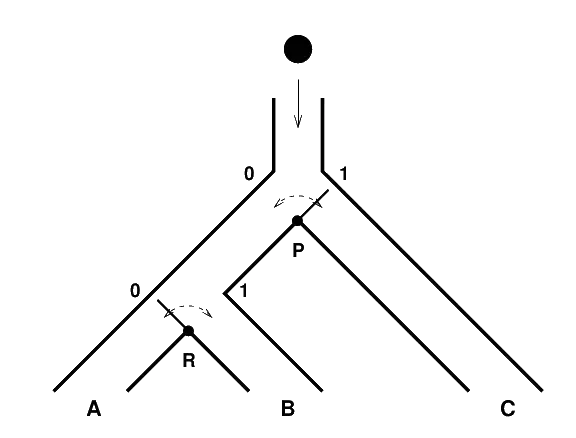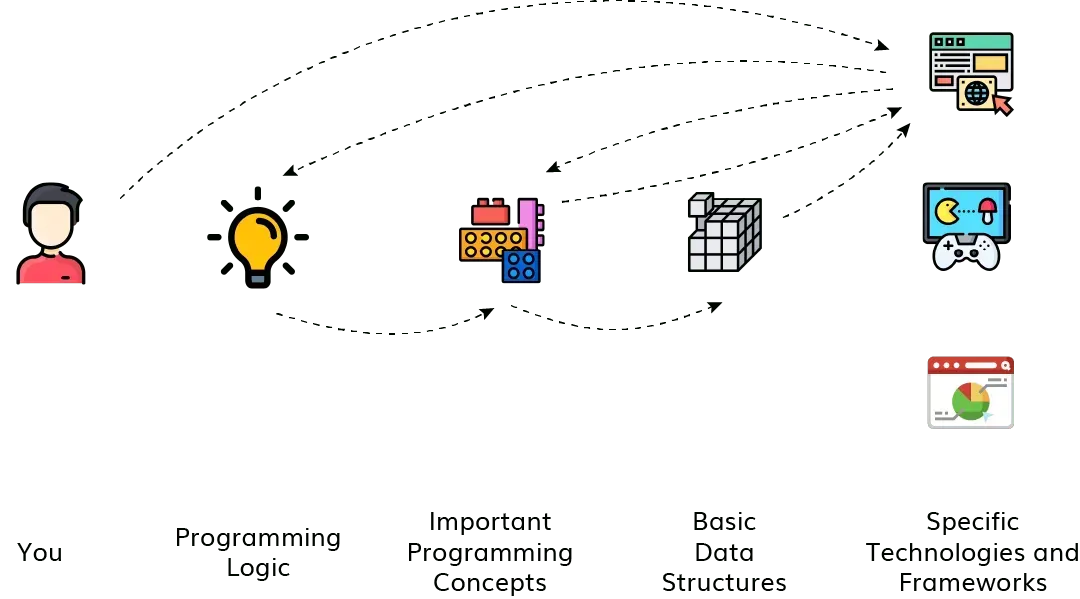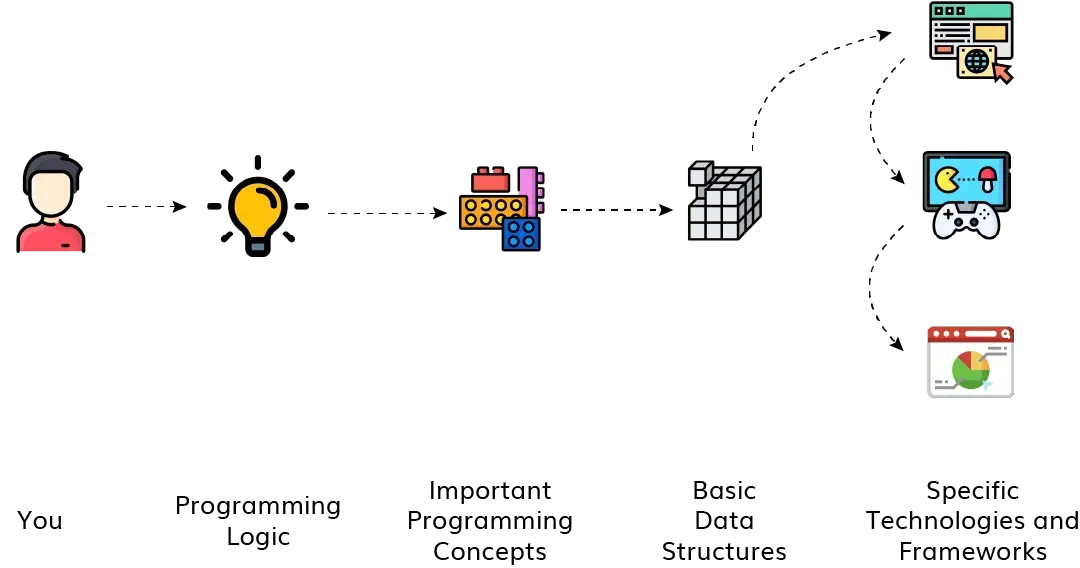# What is the best way to learn programming logic?

Computer Science

Hello,

Many people ask me how do I get started with computer programming? and the immediate next question is what is the first programming language they should learn? Maybe you are here to find out precisely the answers to these questions, but let’s start before that.

The first question someone that want to get started with programming should ask is how do I learn programming logic?

But what is programming logic, you may ask next. We explain.

### Programming Logic

The computer can’t understand English, so you can’t tell him “hey, I want you to do this calculation for me”. Then, we need a way to communicate with the computer, A way that allows us to give instructions to be computed.

These instructions need syntax and semantic meaning. The programming language is the syntax; it is the way that you write your ideas. But how can you write your ideas if you don’t know what to write?

This is where programming logic comes into play. It is the semantic, a way to organize your ideas so that the computer understands. It often gets confused with another term: algorithm.

### Algorithm

An algorithm is a step-by-step instruction to accomplish a task. In programming, it is a set of instructions that tells the computer what to do.

So is programming logic and algorithm the same thing? Let’s see an example to make things clear.

Given the following image (see Figure 1), we want to know where the ball will fall based on the states of P and R. If P is in the status 0, we know that the ball will fall at C.Figure 1

Without noticing, you already started to think about the solution to the task. Let’s start listing some facts.

1. When P = 0, the ball will always fall in C
2. When P = 1, the state of R matters.
3. If P = 1 and R = 0 it will fall in B.
4. If P = 1 and R = 1 it will fall in A.

Great! We already used our programming logic with no effort. We thought of a solution to the problem in a way that we can transcribe it to an algorithm. Let’s do that.

If P = 0:
Ball falls in C
If P = 1:
If R = 0:
Ball falls in B
If R = 1:
Ball falls in A


At this point, we organized our ideas in a way that the computer can understand (programming logic) and wrote step-by-step instructions that if someone follows will give the right answer to our problem (algorithm).

Next, we need to write it in a way the computer can actually process. So we need a programming language. We got the semantic ready. Now let’s get the syntax.

### Picking a programming language

Now it is time. We need to pick a programming language to start. A lot of people recommend starting with the language that is professionally used by something that you like. So if you…

1. … like websites, you should start with Javascript.
2. … like data science or artificial intelligence, you should start with Python.
3. … dream to work in a traditional company, start with Java.

What is the problem with this approach? What looks like the main benefit is actually the main drawback of this approach. People want to save time and become good programmers as fast as possible. So they want to learn one programming language, one tool and conquer the world!!!

It does not work that way.

Choosing to skip the basic concepts is like choosing to start a series of books from the third volume. You may be able to put the pieces together and get an overall idea about what is going on, but you will never understand the big picture. Just take a look at Figure 2; it is easy to see why this does not work.Figure 2 : Common and inefficient way to learn programming

We recommend beginners to start learning:

• How programming languages work in general;
• How a variable is stored in computer memory;
• What are the different kinds of data structures and when to use them;
• and many more basic concepts.

Now compare Figure 2 with Figure 3. We can clearly see that the later is way more organized and efficient.Figure 3 : Efficient way to learn programming

This is the way we teach here at Neps!

A perfect place to get started is our free Introduction to Programming course. If you are a complete beginner and don’t even know the parts of a computer, what is software, and what is an algorithm, we have an even more basic course called Computer 101 where you will learn all this.

I wish you the best in your journey. If you have any doubt, join us on our Discord channel, you will be very welcomed there.